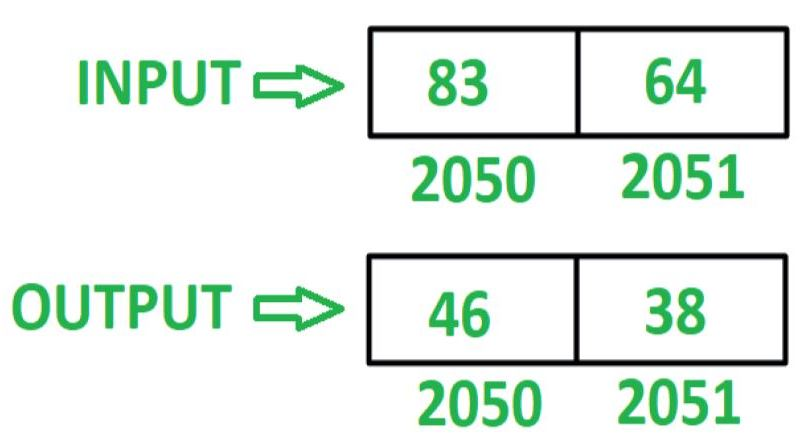Open In App

# 8085 program to reverse 16 bit number

Problem – Write an assembly language program in 8085 microprocessor to reverse 16 bit number.

Example – Assume 16 bit number is stored at memory location 2050 and 2051.Algorithm –

1. Load contents of memory location 2050 in register L and contents of memory location 2051 in register H
2. Move contents of L in accumulator A
3. Reverse the contents of A by executing RLC instruction 4 times
4. Move the contents of A in L
5. Move the contents of H in A
6. Reverse the contents of A by executing RLC instruction 4 times
7. Move the contents of L in H
8. Move the contents of A in L
9. Store the content of L in memory location 2050 and contents of H in memory location 2051

Program –

2000LHLD 2050L <- M, H <- M
2003MOV A, LA <- L
2004RLCRotate accumulator content left by 1 bit without carry
2005RLCRotate accumulator content left by 1 bit without carry
2006RLCRotate accumulator content left by 1 bit without carry
2007RLCRotate accumulator content left by 1 bit without carry
2008MOV L, AL <- A
2009MOV A, HA <- H
200ARLCRotate accumulator content left by 1 bit without carry
200BRLCRotate accumulator content left by 1 bit without carry
200CRLCRotate accumulator content left by 1 bit without carry
200DRLCRotate accumulator content left by 1 bit without carry
200EMOV H, LH <- L
200FMOV L, AL <- A
2010SHLD 2050M <- L, M <- H
2013HLTEND

Explanation – Registers A, H, L are used for general purpose.

1. LHLD 2050: loads contents of memory location 2050 in L and 2051 in H.
2. MOV A, L: moves content of L in A.
3. RLC: shift the content of A left by one bit without carry. Repeat the current instruction 4 times so that contents of A get reversed.
4. MOV L, A: moves the content of A in L.
5. MOV A, H: moves the content of H in A.
6. RLC: shift the content of A left by one bit without carry. Repeat the current instruction 4 times so that contents of A get reversed.
7. MOV H, L: moves the content of L in H.
8. MOV L, A: moves the content of A in L.
9. SHLD 2050: stores the content of L in 2050 and H in 2051.
10. HLT: stops executing the program and halts any further execution.